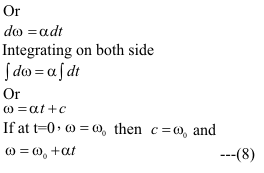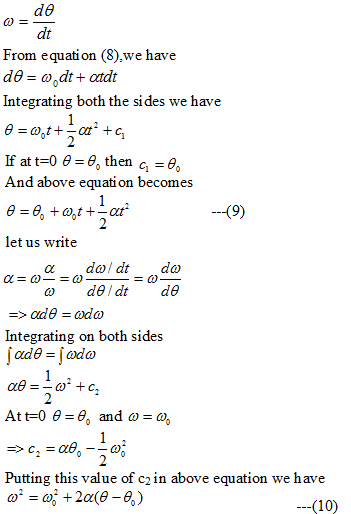# Rotation with Constant Angular Acceleration

## Rotation with constant angular acceleration

• We have already studied motion with constant acceleration while studying translational motion
• Now we will study the rotational motion with constant angular acceleration
• when a rigid body rotates with constant acceleration we have• Thus we have• Again we have angular velocity• Equation (8) ,(9) and (10) are the equation of motion with constant angular acceleration

Question 1. A wheel initially has an angular velocity of 18 rad/s. It has a constant angular acceleration of 2.0 rad/s2 and is
slowing at first. What time elapses before its angular velocity is 18 rad/s in the direction opposite to its initial
angular velocity?

a 3 sec
b 6 sec
c 18 sec
d none of these

Solution 11

ω0=18
ω=-18

angular acceleration(α)=-2

Now

ω=ω0+αt
or t=18